Courses

# Test: Reaction Mechanism- 2

## 30 Questions MCQ Test Organic Chemistry | Test: Reaction Mechanism- 2

Description
This mock test of Test: Reaction Mechanism- 2 for Chemistry helps you for every Chemistry entrance exam. This contains 30 Multiple Choice Questions for Chemistry Test: Reaction Mechanism- 2 (mcq) to study with solutions a complete question bank. The solved questions answers in this Test: Reaction Mechanism- 2 quiz give you a good mix of easy questions and tough questions. Chemistry students definitely take this Test: Reaction Mechanism- 2 exercise for a better result in the exam. You can find other Test: Reaction Mechanism- 2 extra questions, long questions & short questions for Chemistry on EduRev as well by searching above.
QUESTION: 1

Solution:
QUESTION: 2

Solution:
QUESTION: 3

### 1, 3-Dichloropropane one reaction with Zn and NaI gives:

Solution:
QUESTION: 4

In the following reactions:

(a)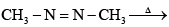(b)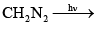(c)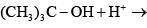(d)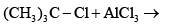The reaction intermediate formed will be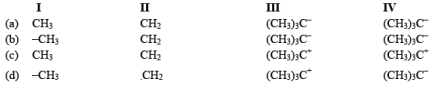Solution:
QUESTION: 5

The intermediate in the reaction of m-bromoanisole with sodamide in liquid amnonia has:

Solution:
QUESTION: 6

Which one is least stable carbanion:

Solution:
QUESTION: 7

Which of the alkyl halides undergoes most readily for nucleophilic substitution reaction:

Solution:
QUESTION: 8

The reaction of ethanolic KOH on 1, 1-dichloropropane gives:

Solution:
QUESTION: 9

Which reactive intermediate is believed to be part of the reaction shown: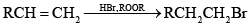Solution:
QUESTION: 10

In this transformat ion,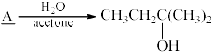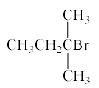What is the best structure for A?

Solution:
QUESTION: 11

The major product formed in the following reaction is: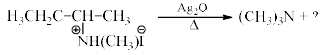Solution:
QUESTION: 12

Which of the following statements does not fit in the criteria of E2 reactions?

Solution:
QUESTION: 13

The major product formed in the following reaction is: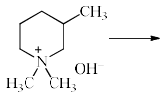Solution:
QUESTION: 14

The electrophilic aromatic substitution proceeds through an intermediate:

Solution:
QUESTION: 15

The relative rates of nitration of R – C6H5, where R = CH3, NO2, OH and Cl is:

Solution:
QUESTION: 16

Which of the following statements is not true for the E2 reactions?

Solution:
QUESTION: 17

Which of the following carbocations is the most stable?

Solution:
QUESTION: 18

Which of the following elimination reactions will give 1-butene as the major product?

Solution:
QUESTION: 19

Which of the following haloalkanes will undergo hydrolysis most readily?

Solution:
QUESTION: 20

The reactant, M in the reaction below: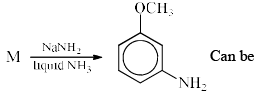Solution:
QUESTION: 21

Arrange the following three chlorides in decreasing order of SN1 reactivity

(I) CH3CH2CH2Cl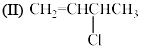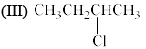Solution:
QUESTION: 22

Arrange the following carbanions in order of their decreasing stability: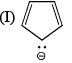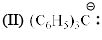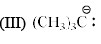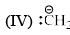Solution:
QUESTION: 23

The following reaction proceeds through: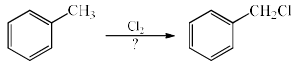Solution:
QUESTION: 24

In the reaction sequence,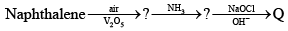Solution:
QUESTION: 25

Arrange the compounds in order of decreasing reactively toward electrophilic substitution: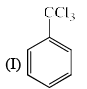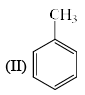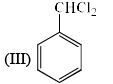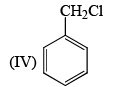Solution:
QUESTION: 26

The major product expected from the following reactionis?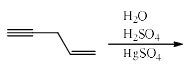Solution:
QUESTION: 27

The rate of decarboxylation of isomeric carboxylic acids is:

Solution:
QUESTION: 28

An intermediate in racemization of (R)-3-phenyl-2-butanone is: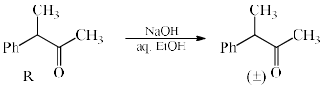Solution:
QUESTION: 29

The intermediate involved in Curtius rearrangement is:

Solution:
QUESTION: 30

In the SN1 solvolysis of the following primary alky chlorides in aqueous ethanol, the order of decreasing reactivity is: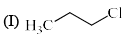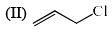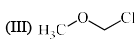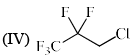Solution: